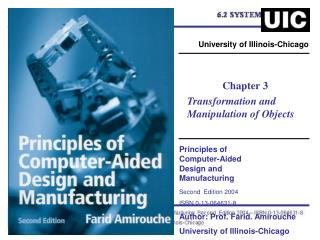DownloadDownload PresentationPrinciples of Computer-Aided Design and Manufacturing Second Edition 2004 ISBN 0-13-064631-8 Author: Prof. Farid. Am

# Principles of Computer-Aided Design and Manufacturing Second Edition 2004 ISBN 0-13-064631-8 Author: Prof. Farid. Am

Download Presentation## Principles of Computer-Aided Design and Manufacturing Second Edition 2004 ISBN 0-13-064631-8 Author: Prof. Farid. Am

- - - - - - - - - - - - - - - - - - - - - - - - - - - E N D - - - - - - - - - - - - - - - - - - - - - - - - - - -
##### Presentation Transcript

1. University of Illinois-Chicago Chapter 3 Transformation and Manipulation of Objects Principles of Computer-Aided Design and Manufacturing Second Edition 2004 ISBN 0-13-064631-8 Author: Prof. Farid. Amirouche University of Illinois-Chicago

2. 3.1 Introduction Motorcycle Engine Design

3. 3D Detailed Building Layouts

4. Automobile Body Display

5. An Auxiliary View of a Building

6. 3.2 Transformation Matrix 3.3 2D Transformation

7. Where = Shear along x-direction. = Shear along y-direction

8. 3.4 Arbitrary Rotation about the Origin Counterclockwise rotation of x and y to obtain and

9. x1 Where is the rotation matrix.

10. 3.5Rotation by Different Angles Arbitrary rotation of axes x and y

11. 3.6 Concatenation 3.7 2D Translation

12. R= R= Reverse the order of the 2 matrices

13. 3.8 Projection onto a 2D Plane R1 = where X*=x, y*=y

14. 3.9 Overall Scaling R= . = An example for overall scaling of an 2D object

15. 3.10 Rotation about an Arbitrary Point • Example Rotation of an Object about an Arbitrary Point in 2D Let C describe an object or configuration of some geometry, where C is an array of data-point coordinates.

16. Solution: + - - [R] = Rotation about arbitrary point.

17. Example Uniform Scaling in 2D Find the transformation matrix that would produce rotation of the geometry about point A, s shown in Figure 3.11(a), followed by a uniform scaling of the geometry down to half its original size.

18. Solution: Step 1: Place the points into a matrix. Step 2: Translate point A to the origin, that is, -2- along the x-axis and -10 along the y Axis, as shown in Figure 3.11(b). Step 3: Rotate the object 30 degrees about the z-axis, as shown is Figure 3.12(c). Step 4: Translate point A to its original position as shown in Figure 3.12(d). Step 5: Scale the object to half its original size, as shown is Figure 3.13(e).

19. First step

20. Step 2 and 3

21. Final step

22. 3.11 2D Reflection R= Reflection about y-axis

23. R= Reflection about x-axis

24. Reflection about any arbitrary Point T= R= T1=

25. Reflection about arbitrary point

26. Reflection about arbitrary axis: a) Coordinate transformation to move the line so it passes through o. T1= b) Rotation to make the x-axis align with the given line T2=

27. c) Reflection about the x-axis R= d) Rotation back by an angle  T3= The concatenated matrix expressing the above steps is defined by

28. Reflection about an arbitrary axis y=mx+c Reflection of the object

29. 3.12 3D TRANSFORMATION A trailer with a lower-attachment An energy-fuel vehicle

30. 3.13 3D Scaling (a) Local Scaling:  (b) Overall Scaling : Overall scaling can be achieved by the following transformation matrix where the final coordinates need to be normalized where

31. Overall scaling

32. C = Let R = Then

33. 3D Scaling

34. 3.14 3D Rotation of objects Rx Rotation about x-axis

35. Ry = Rz = Rotation about y-axis Rotation about z-axis

36. Example 3.5: Rotation in 3D Space • The box shown in Figure 3.26(A) will demonstrate rotation about an axis in 3D space. The box shown in the figure is at the initial starting point for all three rotations. The labeled points of the box listed in matrix format (see Sec. 3.3) are used with the transformation rotation matrices, equations (3.37), (3.39), and (3.40), to obtain the new coordinates after rotation (rotations are in a counterclockwise direction in this example)

37. Solution: [C]= Rotation about the x Axis:

38. [C*]= = Rotation about x-axis for 30 degrees.

39. Rotation about the y Axis: [C*]= = Rotation about y-axis for 30 degrees

40. Rotation about the z Axis: [C*] = = Rotation about z-axis for 30 degrees

41. 3.15 3D Reflection and mirror imaging An example for symmetry

42. Reflection about the x-y plane is given: Reflection about the y-z plane is given: Reflection about the x-z plane is given:

43. Example : Building of a Block • Symmetry is the similarity between two objects with respect to a point or a line or a plane. Dimensions of the object with measured from the symmetric plane will be equal for both the object. One object look similar to the mirror image of the other assuming that the central plane acts as a mirror. This concept of symmetry and mirroring are widely used in design and modeling field to reduce model creation time. Use reflection to simplify the creation of the block shown in

44. Coordinate description using a quarter portion of the block.

45. Solution: = Step 1: Establish the transformation matrix to reflect the quarter block about the x-y plane [C*] = CR1 = C

46. Half block obtained by reflection about the xy plane

47. Step 2: Reflect the half portion of the block about the y-z plane Reflection of half portion of the block about yz plane.